# Algebra 1 : How to multiply integers

## Example Questions

### Example Question #31 : How To Multiply Integers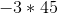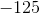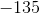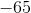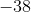Explanation:

When multiplying a negative number and a positive number, our answer is negative. We just multiply normally. Answer is.

### Example Question #32 : How To Multiply Integers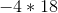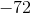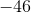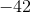Explanation:

When multiplying a negative number and a positive number, our answer is negative. We just multiply normally. Answer is.

### Example Question #33 : How To Multiply Integers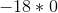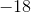Explanation:

Regardless if the number is positive or negative, anything multipled by zero is always zero. So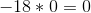### Example Question #34 : How To Multiply Integers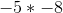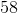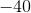Explanation:

When multiplying two negative numbers, the answer is positive. Then multiply normally. Answer is.

### Example Question #35 : How To Multiply Integers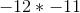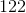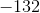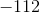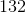Explanation:

When multiplying two negative numbers, the answer is positive. Then multiply normally. Answer is.

### Example Question #36 : How To Multiply Integers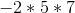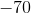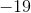Explanation:

When multiplying with more than two integers, we count the number of negative signs. Since there is one, that means our answer is going to be negative. We multiply from left to right. Answer is.

### Example Question #37 : How To Multiply Integers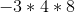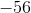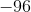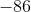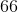Explanation:

When multiplying with more than two integers, we count the number of negative signs. Since there is one, that means our answer is going to be negative. We multiply from left to right. Answer is.

### Example Question #38 : How To Multiply Integers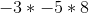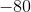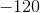Explanation:

When multiplying with more than two integers, we count the number of negative signs. Since there are two, that means our answer is going to be positive. We multiply from left to right. Answer is.

### Example Question #39 : How To Multiply Integers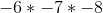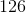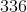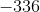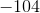Explanation:

When multiplying with more than two integers, we count the number of negative signs. Since there are three, that means our answer is going to be negative. We multiply from left to right. Answer is.

### Example Question #2571 : Algebra 1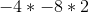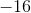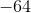When multiplying with more than two integers, we count the number of negative signs. Since there are two, that means our answer is going to be positive. We multiply from left to right. Answer is.